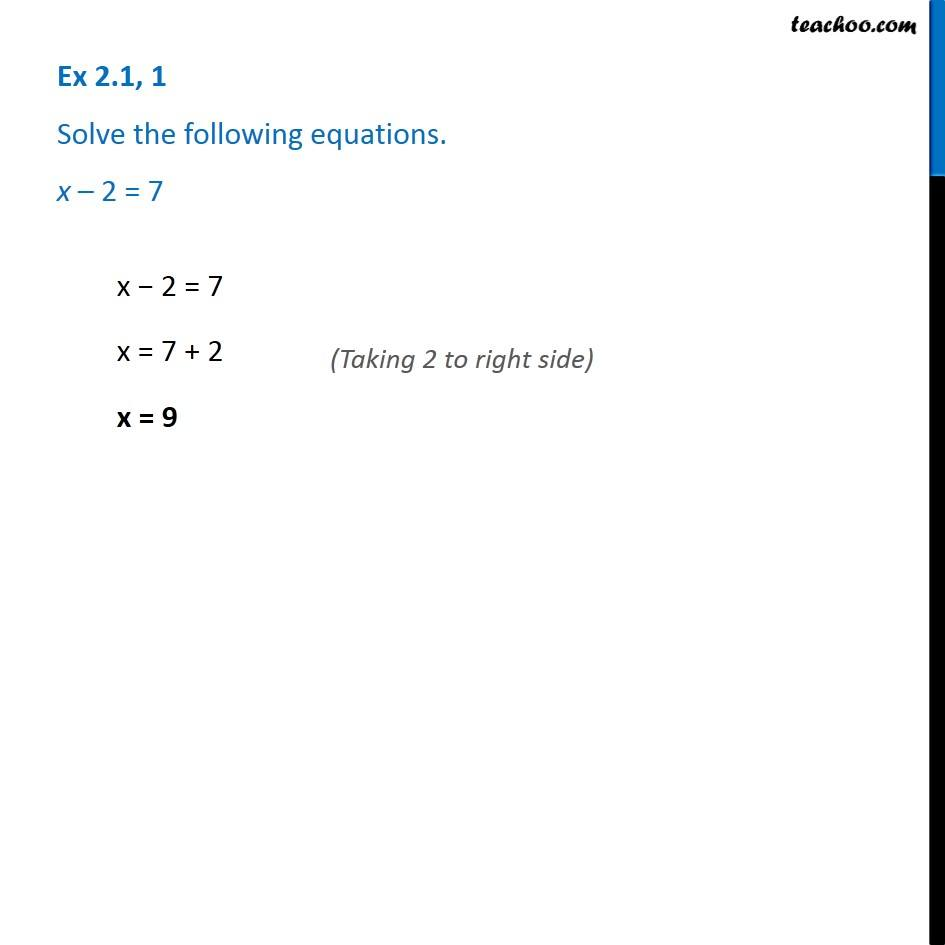1. Chapter 2 Class 8 Linear Equations in One Variable
2. Concept wise
3. Solving Linear Equations - Variables on 1 side

Transcript

Ex 2.1, 1 - Chapter 2 Class 8 - Linear Equations in one variable - NCERT Book Solve the following equations. x - 2 = 7 x - 2 = 7 x = 7 + 2 (Taking 2 to right side) x = 9